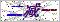订购服务热线： 400-053-8065首页 - 产品中心 - 工业控制传感器/变送器 - 温度传感器/变送器（热电偶、热电阻） - 高温套管温度传感器
 产品目录工业控制传感器/变送器产品中心点击放大
 高温套管温度传感器
 产品型号： 产品报价：产品特点： 高温套管温度传感器，PT100传感器、高温套管式191系列热电偶热电阻采用不锈钢金属外壳封装，内部填充绝缘导热材料密封而成。本产品具有体积小巧、反应灵敏、种类丰富、防水抗震等特点，可广泛用于环境温度、气体温度、液体温度、物体表面温度、冷冻冷藏温度等各种类型的温度测量。
 高温套管温度传感器的详细资料：

zui大测温范围：-55℃～+125℃   测量精度：±0.5℃（-10℃～+85℃）

 类型 P口 0=Pt100      1=Pt1000     5=Pt500     6=Pt10　7=K  8=E　　　　9=指定 温度范围(℃) T口 0=0～80  1=-50～100  2=-50～150  3=-50～200   4=-50～300    9=指定 传感器精度 口 A=A级      B=B级 保护管材质 C口 1=SUS321（默认）   4=SUS304    6=SUS316      9=指定 保护管直径mm D口 4=4   5=5   6=6  8=8   10=10   9=指定 （说明：温度250℃以上时，保护管直径≥10mm） 保护管长度mm G口 40=40 50=50 60=60  80=80  100=100  9=指定 引线长度(mm) L口 1=100   2=200   3=300   5=500  10=1000  30=3000   50=5000 9=指定 引出线缆 X口 2=两线制    3=三线制    4=四线制 引线材质 口 1=聚氯乙烯PVC（-20～80℃）   分有屏蔽层和无屏蔽层两种2=硅橡胶     （-60～150℃）  无屏蔽层3=四氟绕包   （-50～250℃）  有屏蔽层4=云母绕包   （-80～500℃）  无屏蔽层          9=指定 是否配屏蔽层 口 0=无屏蔽层（默认） 1=有屏蔽层 导线末端要求 口 0=无 1=镀锡   2=U型端子  3=针式端子  9=指定 特殊要求 Q口 0=无   9=指定

 如果你对高温套管温度传感器感兴趣，想了解更详细的产品信息，填写下表直接与厂家联系：

### 留言框

• #### 验证码：请输入计算结果（填写阿拉伯数字），如：三加四=7无锡斯洛森测控技术发展有限公司(www.wxckyb.com) 版权所有 热卖产品：露点仪,露点测量仪,在线露点传感器,钢水测温仪,铝材测温仪,在线红外测温仪 总访问量：163412 地址：无锡市崇安区江海东路588号21栋604室 邮编：224200 电话：86-0510-66810836 传真：86-0510-82300399 手机：15852523519 联系人：朱小明 邮箱：2818992521@qq.com GoogleSitemap 技术支持：仪表网 管理登陆 ICP备：苏ICP备14044566号-1